# BDgraph with Simple Examples

#### 2022-12-24

The R package BDgraph provides statistical tools for Bayesian structure learning for undirected graphical models with continuous, count, binary, and mixed data. The package is implemented the recent improvements in the Bayesian graphical models’ literature, including Mohammadi and Wit (2015), Mohammadi et al. (2021), Mohammadi et al. (2017), and Dobra and Mohammadi (2018). Besides, the package contains several functions for simulation and visualization, as well as several multivariate datasets taken from the literature.

Install BDgraph using

install.packages( "BDgraph" )

library( BDgraph )

Here are two simple examples to show how to use the functionality of the package.

# Example 1: Gaussian Graphical Models

Here is a simple example to see the performance of the package for the Gaussian graphical models. First, by using the function bdgraph.sim(), we simulate 200 observations (n = 200) from a multivariate Gaussian distribution with 15 variables (p = 15) and “scale-free” graph structure, as follows

set.seed( 20 )

data.sim = bdgraph.sim( n = 200, p = 15, graph = "scale-free", vis = TRUE )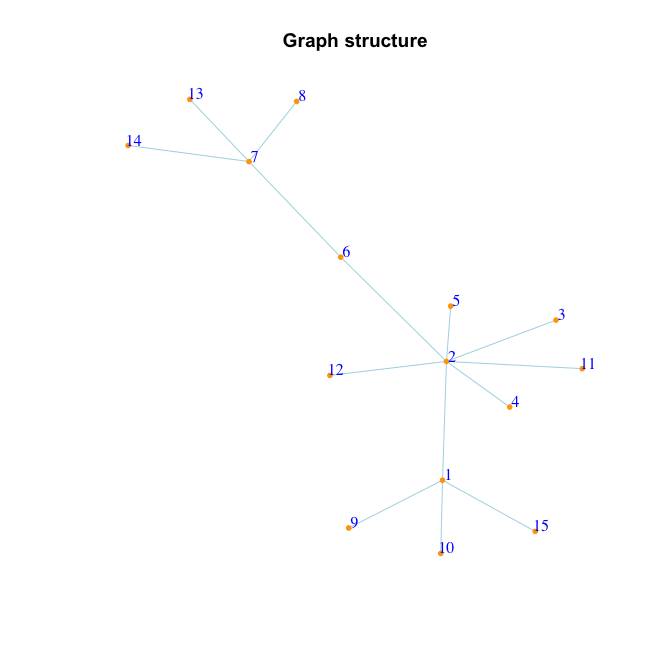Since the generated data are Gaussian, we run the bdgraph() function by choosing method = "ggm", as follows

bdgraph.obj = bdgraph( data = data.sim, method = "ggm", iter = 5000, verbose = FALSE )

To report confusion matrix with cutoff point 0.5:

conf.mat( actual = data.sim, pred = bdgraph.obj, cutoff = 0.5 )
Actual
Prediction  0  1
0 91  4
1  0 10

conf.mat.plot( actual = data.sim, pred = bdgraph.obj, cutoff = 0.5 )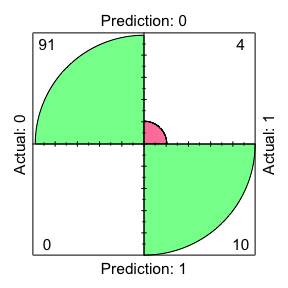To compare the result with the true graph

compare( data.sim, bdgraph.obj, main = c( "Target", "BDgraph" ), vis = TRUE )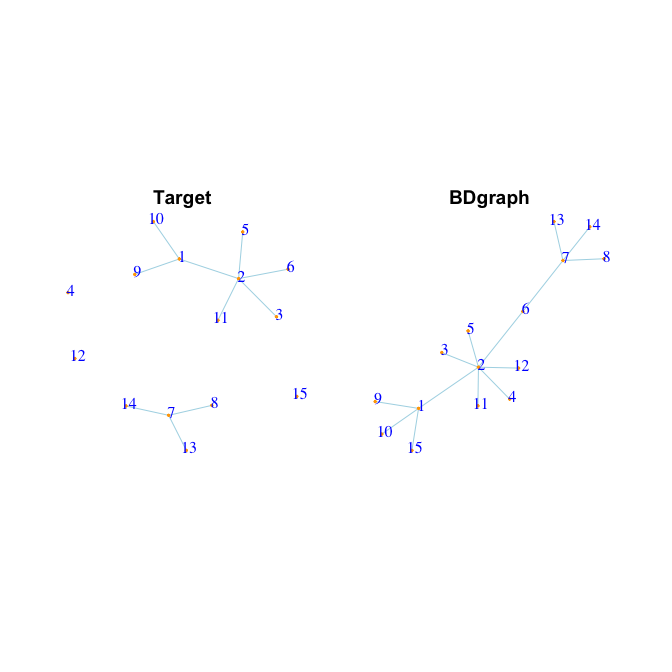Target BDgraph
True Positive      10  10.000
True Negative      95  91.000
False Positive      0   4.000
False Negative      0   0.000
F1-score            1   0.833
Specificity         1   0.958
Sensitivity         1   1.000
MCC                 1   0.827

Now, as an alternative, we run the bdgraph.mpl() function which is based on the GGMs and marginal pseudo-likelihood, as follows

bdgraph.mpl.obj = bdgraph.mpl( data = data.sim, method = "ggm", iter = 5000, verbose = FALSE )

conf.mat( actual = data.sim, pred = bdgraph.mpl.obj )
Actual
Prediction  0  1
0 91  4
1  0 10
conf.mat.plot( actual = data.sim, pred = bdgraph.mpl.obj )We could compare the results of both algorithms with the true graph as follows

compare( list( bdgraph.obj, bdgraph.mpl.obj ), data.sim,
main = c( "Target", "BDgraph", "BDgraph.mpl" ), vis = TRUE )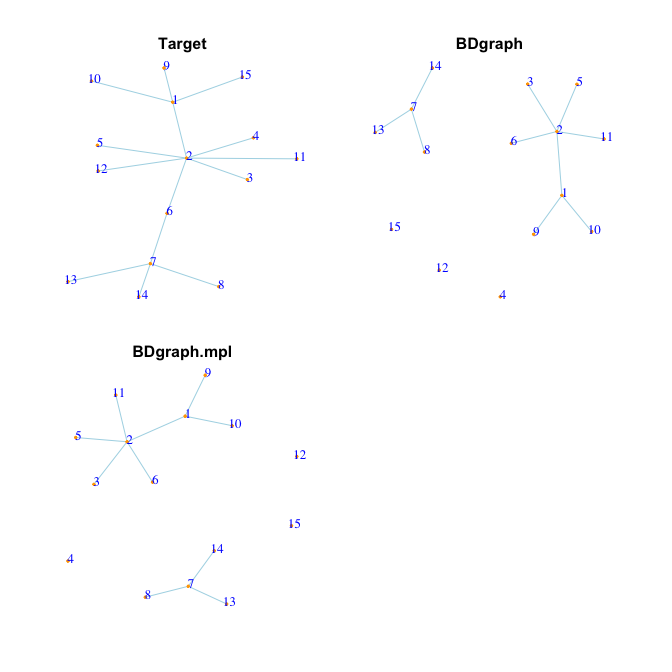Target BDgraph BDgraph.mpl
True Positive      14  10.000      10.000
True Negative      91  91.000      91.000
False Positive      0   0.000       0.000
False Negative      0   4.000       4.000
F1-score            1   0.833       0.833
Specificity         1   1.000       1.000
Sensitivity         1   0.714       0.714
MCC                 1   0.827       0.827

To see the performance of the BDMCMC algorithm we could plot the ROC curve as follows

plotroc( list( bdgraph.obj, bdgraph.mpl.obj ), data.sim, cut = 200,
labels = c( "BDgraph", "BDgraph.mpl" ), color = c( "blue", "red" ) )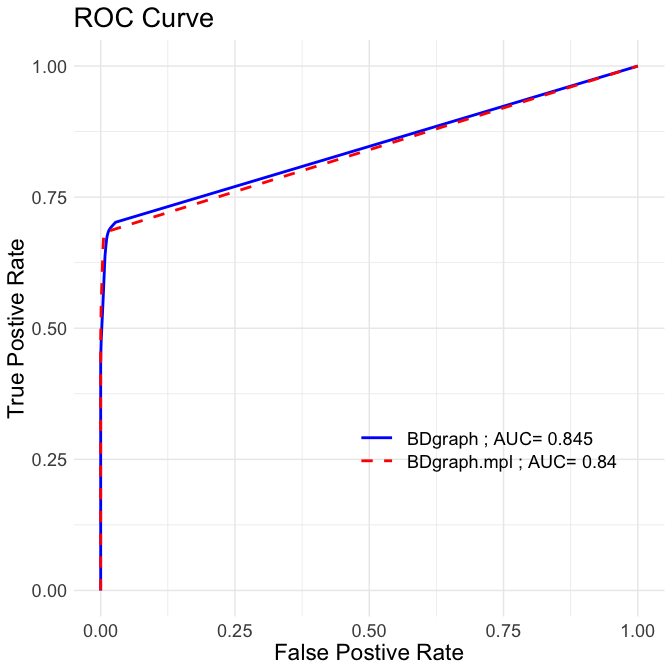# Example 2: Gaussian Copula Graphical Models

Here is a simple example to see the performance of the package for the mixed data using Gaussian copula graphical models. First, by using the function bdgraph.sim(), we simulate 300 observations (n = 300) from mixed data (type = "mixed") with 10 variables (p = 10) and “random” graph structure, as follows

set.seed( 2 )

data.sim = bdgraph.sim( n = 300, p = 10, type = "mixed", graph = "random", vis = TRUE )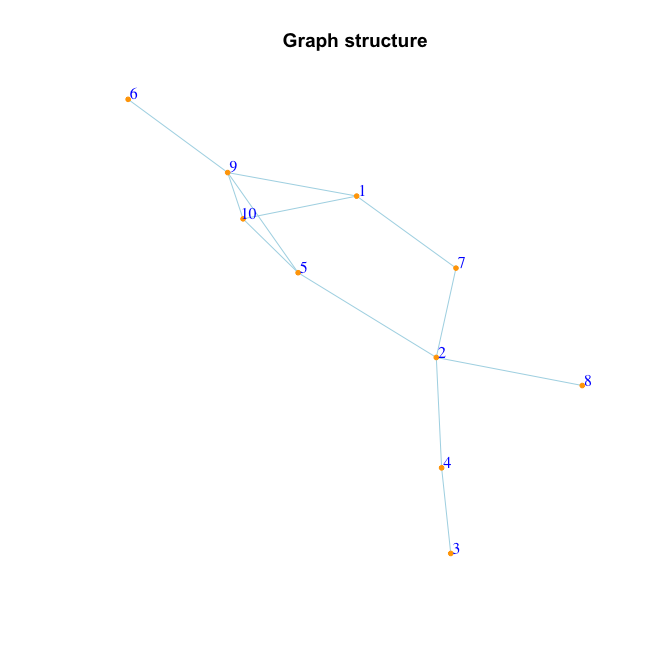Since the generated data are mixed data, we are using run the bdgraph() function by choosing method = "gcgm", as follows:

bdgraph.obj = bdgraph( data = data.sim, method = "gcgm", iter = 5000, verbose = FALSE )

To compare the result with the true graph, we could run

compare( bdgraph.obj, data.sim, main = c( "Target", "BDgraph" ), vis = TRUE )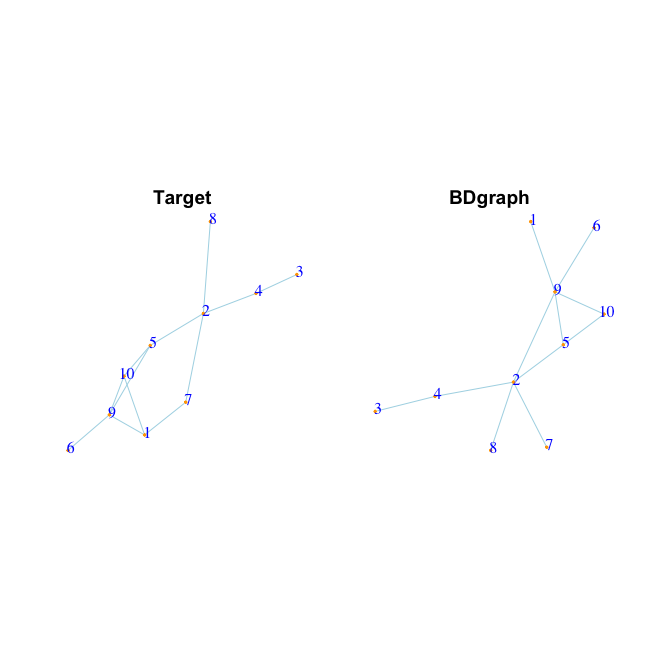Target BDgraph
True Positive      12  10.000
True Negative      33  32.000
False Positive      0   1.000
False Negative      0   2.000
F1-score            1   0.870
Specificity         1   0.970
Sensitivity         1   0.833
MCC                 1   0.826
plotroc( bdgraph.obj, data.sim, labels = "BDgraph", color = "blue" )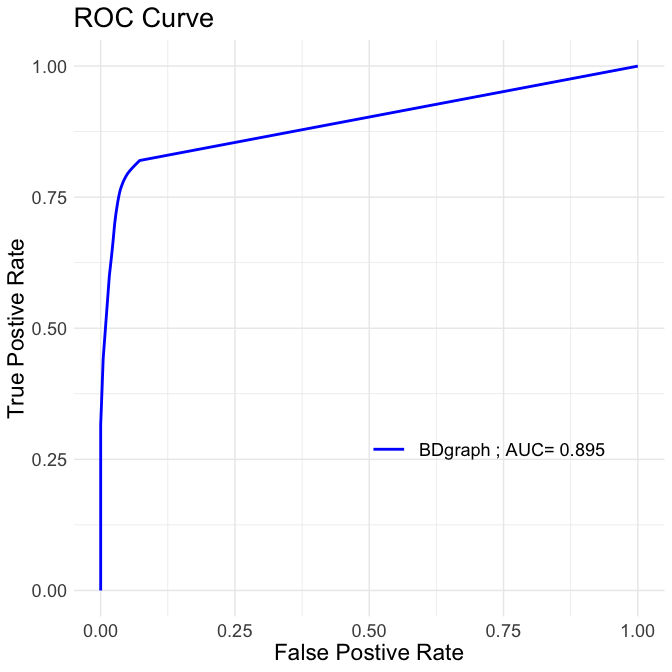For more examples see Mohammadi and Wit (2019).